• +91 9971497814
• info@interviewmaterial.com

# RD Chapter 24- Measures of Central Tendency Ex-VSAQS Interview Questions Answers

### Related Subjects

Question 1 : If the ratio of mean and median of a certain data is 2:3, then find the ratio of its mode and mean

Given that the ratio of mean and median of a certain data is 2:3. That is,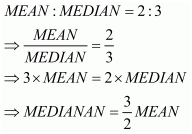We know that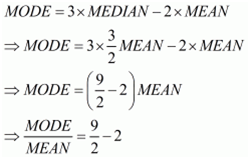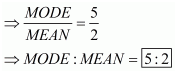Question 2 : If the ratio of mode and median of a certain data is 6 : 5, then find the ratio of its mean and median.

Given that the ratio of mode and median of a certain data is 6:5. That is,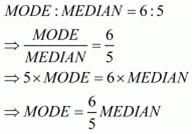We know that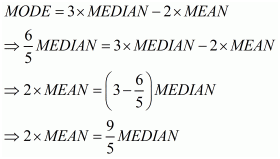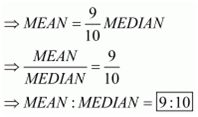Question 3 : If the mean of x + 2, 2x + 3, 3x + 4, 4x + 5 is x + 2, find x.

The given data is x+2, 2x+3, 3x+4, 4x+5. They are four in numbers.
The mean is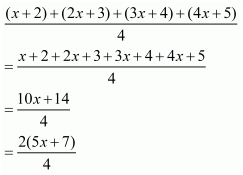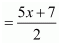But, it is given that the mean is x+2. Hence, we have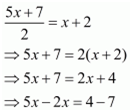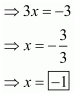Question 4 : The arithmetic mean and mode of a data are 24 and 12 respectively, then find the median of the data.

Given that the arithmetic mean and mode of a data are 24 and 12 respectively. That is,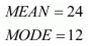We have to find median
We know that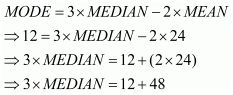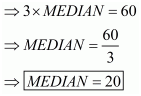Question 5 : If the difference of mode and median of a data is 24, then find the difference of median and mean.

Given that the difference of mode and median of a data is 24. That is,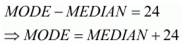We have to find the difference between median and mean
We know that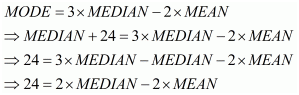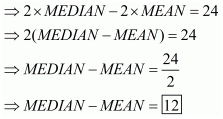Question 6 : If the median of scores x2,x3,x4,x5x2,x3,x4,x5 and x6x6 (where x > 0) is 6, then find the value of x6x6.

Given that the median of the scores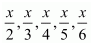, where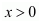is 6. The number of scores n is 5, which is an odd number. We have to find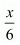Note that the scores are in descending order. Hence the median is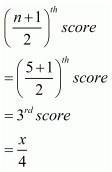But, it is given that the median is 6. Hence, we have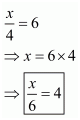Question 7 : If the mean of 2, 4, 6, 8, x, y is 5, then find the value of x + y.

The given data is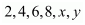. They are 6 in numbers.
The mean is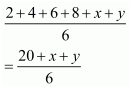But, it is given that the mean is 5. Hence, we have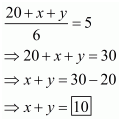Question 8 : If the mode of scores 3, 4, 3, 5, 4, 6, 6, x is 4, find the value of x.

The given data is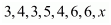.
The mode is the value which occur maximum number of times, that is, the mode has maximum frequency. If the maximum frequency occurs for more than 1 value, then the number of mode is more than 1 and is not unique.
Here it is given that the mode is 4. So, x must be 4, otherwise it contradicts that the mode is 4. Hence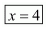Question 9 : If the median of 33, 28, 20, 25, 34, x is 29, find the maximum possible value of x.

The given data is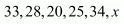. The total number of values is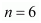, is an even number. Hence the median depends on the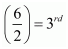observation and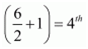observation.
Since we have to find the maximum possible value of x.So we must put it in the 4th position when ordering in ascending order.
Arranging the data in ascending order, we have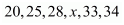Hence, the median is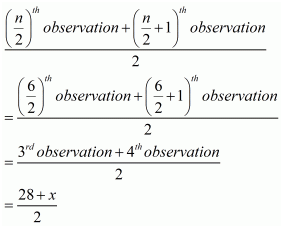Here it is given that the median is 29. So, we have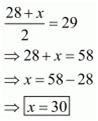Question 10 : If the median of the scores 1, 2, x, 4, 5 (where 1 < 2 < x < 4 < 5) is 3, then find the mean of the scores.

The given data is 1, 2, x, 4 and 5. Since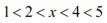, the given data is already in ascending order.
Here, the number of observation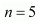, which is an odd number.
Hence, the median is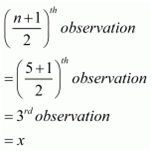Here, it is given that the median is 3. Hence, we have .
The mean is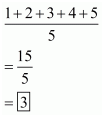Todays Deals### RD Chapter 24- Measures of Central Tendency Ex-VSAQS Contributorskrishan

Name:
Email:

# Latest News# 9000 interview questions in different categories